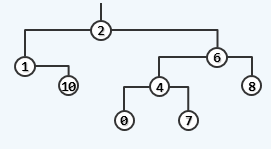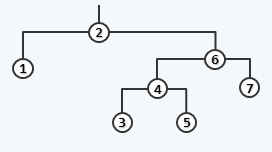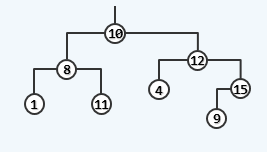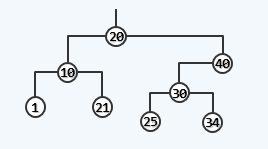HR
【单选题】Which of the following is a BST? 以下哪项是二叉搜索树?
A.B.C.D.手机使用分享复制链接新浪微博分享QQ微信扫一扫反馈A.

B.

A.

B.

A.

B.

C.

D.

A.

B.

C.

D.

A.

B.

C.

D.

E.

A.

B.

C.

D.

A.
5
B.
8
C.
10
D.
12
PE线截面选择：当与相线材质相同时，应满足：16mm2＜相截面≤ 35mm2时， PE线截面为（ ）。
A.
16mm2
B.

C.

D.
4mm2

A.

B.

A.

B.

A.
1/10加0.9米
B.
1/9加0.6米
C.
1/10加0.6米
D.
1/8加0.6米

A.

B.

C.

D.

A.

B.

C.

D.

A.

B.

A.

B.

A.

B.

A.

B.

A.

B.

A.
1KV
B.
3KV
C.
6KV
D.
10KV

A.
B.
C.
D.

A.

B.

C.

D.

A.

B.

A.

B.

C.

D.

A.

B.

PE线截面选择：当与相线材质相同时，应满足：相线截面＞ 35mm2时， PE线截面为（ ）。
A.
16mm2
B.

C.

D.
4mm2

A.

B.

C.

D.

A.
1000V
B.
500V
C.
380V
D.
250V

A.

B.

C.

D.

E.

A.

B.

A.

B.

C.

D.

A.

B.

C.

D.

A.
5
B.
5.5
C.
6
D.
6.5

A.

B.

C.

A.
380V，500V
B.
400V，500V
C.
1000V，1500V
D.
800V，1200V

A.
15
B.
20
C.
25
D.
30
PE线必须在每个电箱处做重复接地。
A.

B.

A.

B.

A.

B.

A.

B.

A.
1.0
B.
0.9
C.
0.8
D.
0.6

A.

B.

C.

D.

A.

B.

A.

B.

A.
5；50
B.
8；50
C.
10；80
D.
4；50

A.

B.

C.

D.

A.

B.

C.

D.

E.

A.

B.

C.

D.

A.

B.

C.

D.

A.

B.

A.

B.

C.

1 牛顿（ N） =1 公斤力（ kgf ）。
A.

B.

A.

B.

A.
1欧姆
B.
2欧姆
C.
3欧姆
D.
4欧姆

A.

B.

C.

D.

A.

B.

C.

D.

A.

B.

C.

D.

A.
5
B.
10
C.
20
D.
30

A.

B.

A.

B.

A.

B.

A.

B.

A.

B.

C.

D.

E.

A.
1.2～1.5
B.
2～2.5
C.
3～3.5
D.
5.0

A.

B.

A.

B.

A.

B.

C.

A.

B.

C.

D.

A.
16平方毫米
B.
18平方毫米
C.
22平方毫米
D.
20平方毫米

A.

B.

A.

B.

A.
2
B.
1.5
C.
1.0
D.
0.5

A.

B.

C.

A.
0.5-1
B.
1.1-1.2
C.
1.2-1.3
D.
1.3-1.4

A.
：2.2
B.
：2.5
C.
：2.8

A.

B.

A.

B.

A.

B.

C.

D.

E.

A.

B.

C.

D.

A.

B.

A.

B.

C.

D.

A.
BX
B.
RVV
C.
VV
D.
ZQ

A.

B.

A.

B.

C.

D.

E.

A.

B.

A.

B.

A.

B.

A.

B.

A.
300.0
B.
400.0
C.
500.0
D.
600.0

A.

B.

A.

B.

A.

B.

A.

B.

C.

D.

A.

B.

C.

D.

A.
24V
B.
36V
C.
12V

A.

B.

A.

B.

（ ）须和按钮开关、继电器等配合使用，能远距离、自动、频繁地通断交直流电路，没有过载短路保护，但能实现失压和欠压保护。
A.

B.

C.

D.

A.
TT；
B.
TN—C；
C.
TN；
D.
TN—S

A.

B.

C.

D.

E.

A.

B.235%